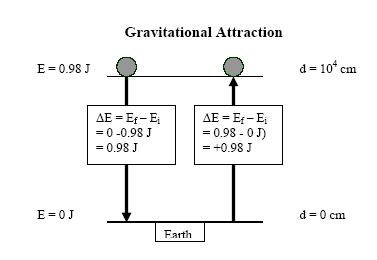# Gravitational and Electrostatic Potential Energy

$$\newcommand{\vecs}{\overset { \rightharpoonup} {\mathbf{#1}} }$$ $$\newcommand{\vecd}{\overset{-\!-\!\rightharpoonup}{\vphantom{a}\smash {#1}}}$$$$\newcommand{\id}{\mathrm{id}}$$ $$\newcommand{\Span}{\mathrm{span}}$$ $$\newcommand{\kernel}{\mathrm{null}\,}$$ $$\newcommand{\range}{\mathrm{range}\,}$$ $$\newcommand{\RealPart}{\mathrm{Re}}$$ $$\newcommand{\ImaginaryPart}{\mathrm{Im}}$$ $$\newcommand{\Argument}{\mathrm{Arg}}$$ $$\newcommand{\norm}{\| #1 \|}$$ $$\newcommand{\inner}{\langle #1, #2 \rangle}$$ $$\newcommand{\Span}{\mathrm{span}}$$ $$\newcommand{\id}{\mathrm{id}}$$ $$\newcommand{\Span}{\mathrm{span}}$$ $$\newcommand{\kernel}{\mathrm{null}\,}$$ $$\newcommand{\range}{\mathrm{range}\,}$$ $$\newcommand{\RealPart}{\mathrm{Re}}$$ $$\newcommand{\ImaginaryPart}{\mathrm{Im}}$$ $$\newcommand{\Argument}{\mathrm{Arg}}$$ $$\newcommand{\norm}{\| #1 \|}$$ $$\newcommand{\inner}{\langle #1, #2 \rangle}$$ $$\newcommand{\Span}{\mathrm{span}}$$$$\newcommand{\AA}{\unicode[.8,0]{x212B}}$$

The foundation for understanding bonding in chemistry is a fairly simple concept: Potential energy. Potential energy (Ep) is the energy which one or more bodies have because of their position.

==Gravitational Potential Energy==IWC replica Réplica de reloj It's simple because we can understand it in terms of the common experience of lifting an object. Audemars Piguet Royal According to the law of conservation of energy, the muscle energy expended for a bird to rise from the surface of the earth to 100 m cannot be created or destroyed, so it must be converted to potential energy, Ep. The bird's Ep is large at 100 m height. In other words, the bird has a large amount of potential energy that can then be converted to other forms. As the bird descends, Ep is released, or better, converted to other forms of energy, like wind turbulance or heat in the atmosphere. The Potential energy is calculated with the formula:

$\text{E}_p = \text{mgh}\label{1}$

where g is the acceleration of gravity (9.8 m s-2, m is the mass, and h is the height of the object.

According to equation $$\ref{1}$$, the PE must be zero when the height is zero. For a mass of 1 g (1/5 the mass of a nickel) at a height of 100 m:

$\text{E}_p = \text{mgh} = \text{10}^{-3} \text{kg} \times \text{9.8 m s}^{-2} \times \text{10}^2 \text{m} = \text{0.98 J}\nonumber$Figure $$\PageIndex{1}$$ Gravitational Attraction

When the object falls, it releases energy, and the energy is given a negative sign to show that it's lost by the object. It's change in energy, "ΔE", is always calculated as the final energy (Ep,f) minus the initial energy (Ep,i).

$\Delta \text{E}_p = \text{E}_{p,f} - \text{E}_{p,i} = \text{0 J - 0.98 J} = -\text{0.98 J}\nonumber$

## Electrostatic or "Coulombic" Potential Energy

Electrostatic forces between electrons and nuclei lead to chemical bonding, and the picture is a little different because these may be either forces of attraction or repulsion. Because of the electrostatic force of attraction or repulsion between them, two charged particles will vary in energy as we alter the distance between them.

Opposite (+ and -) charges attract, just like the earth and any object, so the potential energy increases as we put effort in to separate the charges. Once they're separated, they will "fall" together, releasing Ep.

But like charges (+ and +, or - and -) repel, so it takes effort to push them together, and when they're close together they have high potential energy. If they're pushed together and released, they will fly apart, releasing energy.

Because the potential energy of like-charged particles increases as they are brought closer together, while that of opposite-charged particles decreases, it is convenient to assign a value of zero potential energy to two charged particles which are a long distance apart. Bringing one positive and one negative particle together decreases Ep, giving a negative value, indicating that energy is released.Figure $$\PageIndex{2}$$ Electrostatic Attraction and Repulsion

Suppose we have charges of +1 and –1 μC separated by 1 cm, for example. The charges could be separated by hand, and by the time they're 100 m (about a football field) apart, their attractive force would be negligible (it's taken to be zero at infinite separation). Expenditure of muscle energy will be necessary to carry out such a separation. That is, because the charges attract each other, we must do work to pull them apart equivalent to 0.898 J.

$\Delta \text{E}_p = \text{E}_{p,f} - \text{E}_{p,i} = \text{0 J} - \text{E}_{p,i} = +\text{0.898 J}\n\nonumber$

Thus

$-\text{E}_{p,i} = + \text{0.898 J - 0 J}\nonumber$

$\text{E}_{p,i} = -\text{0.898 J}\nonumber$

We say that the 0.898 J is gained by the two charges and stored as potential energy. We can always regain this energy by reversing the process during which it was stored. If the two opposite charges are returned to their original separation of 1 cm, their potential energy will decrease by 0.898 J.

$\Delta \text{E}_p = \text{E}_{p,f} - \text{E}_{p,i} = \text{-0.898 J-0 J} = -\text{0.898 J}\nonumber$

The energy released will appear as kinetic energy, as heat, or in some other form, but it cannot be destroyed.

If we had taken two particles both of which had a charge of +1 μC for our example of potential energy, work would have been required to push them together against their repulsive force. Their potential energy would increase as they were brought together from the ends of a football field, and 0.898 J would be required to move them to a distance of 1 cm apart. Note that in the figure the higher potential energies are given positive values.

$\Delta \text{E}_p = \text{E}_{p,f} - \text{E}_{p,i} = +\text{0.898 J - 0 J} = +\text{0.898 J}\nonumber$

Bringing a pair of positive charges (or a pair of negative charges) closer together increases Ep to a positive value. Bringing one positive and one negative particle together decreases Ep, giving a negative value.

From ChemPRIME: 5.10: Potential Energy

## References

1. the formula is